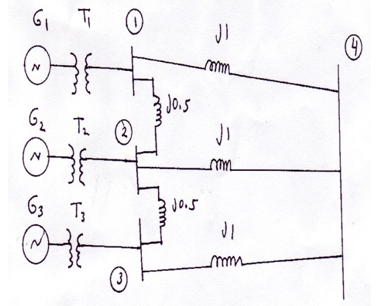### Writing model equation

Assignment Help Physics
##### Reference no: EM1313506

Question: For all generators: Eg = 1\"\"0°, χ1 = j0.25

For all transformers: χ1 = j0.75

[A] Write the Y and Z matrices.
[B] Write the model equation.
[C] For each bus, find V, ρ and Q.### Write a Review